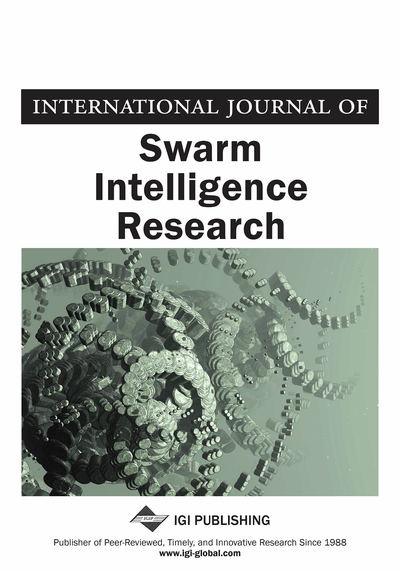# Application of Fireworks Algorithm in Gamma-Ray Spectrum Fitting for Radioisotope Identification

Miltiadis Alamaniotis (Nuclear Engineering Program, University of Utah, Salt Lake City, UT, USA & Applied Intelligent Systems Laboratory, School of Nuclear Engineering, Purdue University, West Lafayette, IN, USA), Chan K. Choi (School of Nuclear Engineering, Purdue University, West Lafayette, IN, USA) and Lefteri H. Tsoukalas (School of Nuclear Engineering, Purdue University, West Lafayette, IN, USA)
DOI: 10.4018/IJSIR.2015040105
Available
\$37.50
No Current Special Offers

## Abstract

Identification of radioisotopic signature patterns in gamma-ray spectra is of paramount importance in various applications of gamma spectroscopy. Therefore, there are several active research efforts to develop accurate and precise methods to perform automated spectroscopic analysis and subsequently recognize gamma-ray signatures. In this work, the authors present a new method for radioisotope identification in gamma-ray spectra obtained with a low resolution radiation detector. The method fits the obtained spectrum with a linear combination of known template signature patterns. Coefficients of the linear combination are evaluated by computing the solution of a single objective optimization problem, whose objective is the Theil-1 inequality coefficient. Optimization of the problem is performed by the Fireworks Algorithm, which identifies a set of coefficients that minimize the Theil-1 value. The computed coefficients are statistically tested for being significantly different than zero or not, and if at least one is found to be zero then the Fireworks Algorithm is used to reiterate fitting using the non-zero templates. Fitting iterations are continued up to the point that no linear coefficients are found to be zero. The output of the method is a list that contains the radioisotopes that have been identified in the measured spectrum. The method is tested on a set of both simulated and real experimental gamma-ray spectra comprised of a variety of isotopes, and compared to a multiple linear regression fitting, and genetic algorithm Theil-1 based fitting. Results demonstrate the potentiality of the Fireworks Algorithm based method, expressed as higher accuracy and similar precision over the other two tested methodologies for radioisotope signature pattern identification in the framework of gamma-ray spectrum fitting.
Article Preview
Top

## Introduction

Gamma spectroscopic analysis is an established and well known framework for identifying isotope signature patterns in a spectrum measured with a radiation detector. Gamma spectroscopy encompasses all those tools that quantitatively analyze obtained energy spectra into their constituents and determine the contribution of each constituent in the measured spectrum (Crouthamel et al, 2013). Though spectroscopy has been widely used in various areas such as radiochemistry and astrophysics for long time, it is not until recently that became of great interest to the areas of nuclear nonproliferation and security, since it may be utilized to detect and prevent potential threats ahead of time (Tsoulfanidis & Landsberger, 2013). To that end, gamma spectroscopy analysis is adopted for analyzing spectra and subsequently identifying radioactive materials that may impose a threat.

A common strategy in gamma radionuclide identification is fitting the measured spectrum with a set of known templates. The templates may be either experimentally measured or simulated spectra characteristic of radioisotopes of interest. Analytically, spectrum fitting is modeled as the linear combination of templates that best matches the measured spectrum (Burr & Hamada, 2009). The degree of matching is quantified as an error measure such as the mean square error or correlation coefficient. Furthermore, each template is multiplied by a linear coefficient that stands for the contribution of the respective isotope in the spectrum. The list of isotope templates associated with non-zero coefficients is identified as the final list of isotopes truly detected in the measured spectrum.

Analytically, isotope spectra and templates are modeled as 1xN dimension matrices (a.k.a. vectors), where N stands for the length of the spectrum. The spectrum length is defined as the number of bins adopted to span the energy range of gamma-ray particles. In other words, an energy spectrum is a histogram where each bin represents an energy interval. The number of bins in a gamma-ray spectrum, which depends on type of detector and detection electronics, takes values equal to 512, 1024, 2048, 4096, 8192, 16384 or 32768. Assuming the population of available templates is L, then spectrum fitting aims at finding the linear combination of the L templates that approximates the measured spectrum (Burr & Hamada, 2009). Getting into details, the fitting process searches for a linear combination of the columns of an NxL matrix such that it is equal or close to the measured vector 1xN, i.e., the measured spectrum, pushing spectrum fitting to fall into the category of “big data problems”.

## Complete Article List

Search this Journal:
Reset
Open Access Articles
Volume 13: 4 Issues (2022): 3 Released, 1 Forthcoming
Volume 12: 4 Issues (2021)
Volume 11: 4 Issues (2020)
Volume 10: 4 Issues (2019)
Volume 9: 4 Issues (2018)
Volume 8: 4 Issues (2017)
Volume 7: 4 Issues (2016)
Volume 6: 4 Issues (2015)
Volume 5: 4 Issues (2014)
Volume 4: 4 Issues (2013)
Volume 3: 4 Issues (2012)
Volume 2: 4 Issues (2011)
Volume 1: 4 Issues (2010)
View Complete Journal Contents Listing